# GRE Math Formula eBookHaving trouble remembering your GRE math formulas on the fly? This free GRE resource should help you!

Although memorizing a GRE math formula (or twelve) isn’t the only way to study for GRE Quant, knowing certain frequently-tested formulas by heart will help you improve your speed on test day. Once you learn these formulas, be sure to practice using them! A little timed practice can go a long way.

Download the Magoosh math formulas eBook and read on to learn a little bit more about what to expect from the math section of the GRE.## GRE Quantitative Reasoning & Useful Math Formulas

The GRE Quantitative Reasoning section tests concepts that you likely learned during sophomore and/or junior year of high school. These concepts DO NOT INCLUDE higher level math like trigonometry, calculus, or geometry proofs. They DO INCLUDE things you’ve probably long forgotten like properties of shapes, integer properties, exponent rules, and word problems.

The thing that takes these concepts to the GRE level is their complexity. Figuring out what each question is asking you to accomplish can be really tricky, whereas the math involved in solving them tends to be fairly straightforward. And you won’t get partial credit for showing your work, so picking the correct answer choice is very important!

Since it may take you longer than you’d like to read and understand what a GRE quant question is asking of you, it’s important that you won’t waste time solving the problem. Knowing which math formulas to use, and then using them quickly and correctly, can really help you do well on GRE quant. Time is of the essence!

## GRE Math Formula Topics

Here is a list of the main concepts and formulas you’ll need to know for the GRE. Each of these concepts is addressed in the Magoosh’s Complete Guide to Math Formulas eBook. In this resource, you’ll also find recommended strategies for how to best use (or not use!) and remember these math formulas on the GRE.

• Arithmetic and Number Properties
• Types of Numbers
• Order of Operations: PEMDAS
• Commutative, Associative, and Distributive Properties
• Prime Numbers
• Factorization
• Divisibility
• Absolute Values
• Fast Fractions
• Percentages
• Ratios
• Powers and Roots
• Exponents
• Roots
• Algebra
• Simplifying Expressions
• Factoring
• Solving Equations
• Geometry
• Angles
• Polygons
• Triangles
• Circles
• Squares
• Rectangles
• Trapezoids
• 3-D Shapes
• Coordinate Geometry
• Lines
• The Distance Formula
• Word Problems
• Distance, Rate, and Time
• Work Rate
• Sequences
• Interest
• Statistics
• Counting
• Probability

Don’t be intimidated! Some of these formulas are easy to remember, and some you’ll still know from your high school days. Focus your time on re-learning the formulas and concepts that are trickiest and newest to you.

## Important GRE Math Formulas

Below are the main math formulas you’ll need for the GRE. I’ve just given a quick overview — a Cliff Notes version — since later on in the post you’ll find the full Math Formula eBook, which contains these formulas and many more. So if you want an in-depth take on this stuff, then head to the eBook at the bottom of the page. If not, look below for your math formula edification.

That said, I should offer a quick word on the temptation of thinking that knowing a formula is the key to automatically answering a question correctly. This is decidedly not the case on the GRE, on which you have to decipher, unscramble, unlock (pick your metaphor!) the question before you can answer it correctly. In other words, the formula is the final step to answering a math question right. (I expound on this in the intro of the eBook below.)

### 1. Area and circumference of a circle

Area: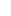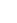, where r is the radius of the circle
Circumference: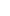, where d is the diameter of the circle

### 2. Volume and surface area of a cube

Surface area: 6s2, where s is the length of a side of the cube
Volume: s3, where s is the length of a side of the cube

### 3. Area of a triangle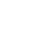,where b is the base and h is the height of the triangle

### 4. Exponent essentials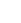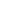=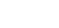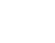=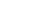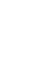=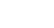### 5. Polynomials and FOIL

(a + b)2 = a2 + 2ab + a2
(ab)2 = a2 − 2ab + a2
(ab)(a + b) = a2b2

FOIL: First Outer Inner Last

### 6. Total degree measure of a polygon

Total degrees = 180(n − 2), where n equals number of sides of polygon

We recommend that you print it out, share it, and start a pile of Magoosh eBooks (General, Vocabulary), that you can study on the go. Then, test out your skills on GRE Quant questions with answers and explanations. Enjoy! Be sure to let us know you like this resource. 🙂

## Author

•Chris Lele is the Principal Curriculum Manager (and vocabulary wizard) at Magoosh. Chris graduated from UCLA with a BA in Psychology and has 20 years of experience in the test prep industry. He's been quoted as a subject expert in many publications, including US News, GMAC, and Business Because. In his time at Magoosh, Chris has taught countless students how to tackle the GRE, GMAT, SAT, ACT, MCAT (CARS), and LSAT exams with confidence. Some of his students have even gone on to get near-perfect scores. You can find Chris on YouTube, LinkedIn, Twitter and Facebook!